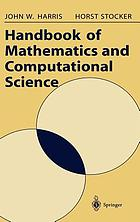Handbook of mathematics and computational science (Book, 2006) [WorldCat.org]Preview this item
Checking...

# Handbook of mathematics and computational science

Author: J Harris; Horst Stöcker New York : Springer, 2006.Print book : English This book gathers thousands of up-to-date equations, formulas, tables, illustrations, and explanations into one invaluable volume. It includes over a thousand pages of mathematical material as well  Read more... (not yet rated) 0 with reviews - Be the first.

## Find a copy in the libraryFinding libraries that hold this item...

## Details

Genre/Form: Handbooks and manualsHandbooks, manuals, etc Book J Harris; Horst Stöcker Find more information about: J Harris Horst Stöcker 0387947469 9780387947464 74134381 Includes index. xxviii, 1028 pages : illustrations ; 25 cm 1. Numerical computation (arithmetics and numerics) -- 2. Equations and inequalities (algebra) -- 3. Geometry and trigonometry in the plane -- 4. Solid geometry -- 5. Functions -- 6. Vector analysis -- 7. Coordinate systems -- 8. Analytic geometry -- 9. Matrices, determinants, and systems of linear equations -- 10. Boolean algebra-application in switching algebra -- 11. Graphs and Algorithms -- 12. Differential calculus -- 13. Differential geometry -- 14. Infinite series -- 15. Integral calculus -- 16. Vector analysis -- 17. Complex variables and functions -- 18. Differential equations -- 19. Fourier transformation -- 20. Laplace and z transformations -- 21. Probability theory and mathematical statistics -- 22. Fuzzy logic -- 23. Neural networks -- 24. Computers -- 25. Tables of integrals. John W. Harris, Horst Stocker.

## Reviews

Editorial reviews

Publisher Synopsis

From the reviews"It is the best text of its type that I have come across to date - an excellent resource for anyone involved in mathematical practice up to and including degree standard (...) it is a Read more...

User-contributed reviewsBe the first.

## Similar Items

### User lists with this item (2)

Confirm this request

You may have already requested this item. Please select Ok if you would like to proceed with this request anyway.

Please sign in to WorldCat

Don't have an account? You can easily create a free account.